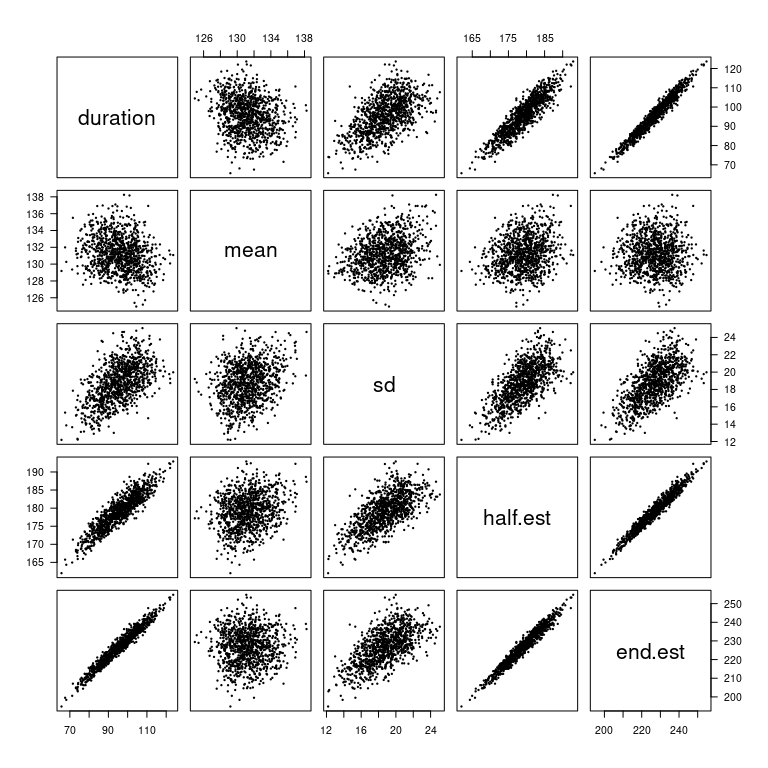# Bootstrap Confidence Intervals for moult

## Introduction

The parameters estimated in a moult analysis are mean start date, duration and standard deviation. The estimates in the moult package are found by maximising a likelihood. One way to construct confidence intervals for these estimates is to first obtain an estimate for the standard error (SE) and then assuming a normal sampling distribution for the estimate and constructing a confidence interval as

$\mbox{estimate} \pm 1.96 \times \mbox{SE(estimate)}.$

In the moult package, SEs are found from the observed information matrix (inverse of the matrix of 2nd derivatives of the log-likelihood function). The above normal confidence interval only works if the sampling distributions of the statistics are close to normal.

A second reason for introducing bootstrap confidence intervals: the standard deviation parameter in the moult package is estimated on the log-scale. It’s SE on the original scale can be approximated (using the Delta method) but finding covariances is much more complicated, e.g. it is difficult to obtain the covariance between start date and standard deviation in start date for both parameters on the original scale.

One solution to solve both of the above problems (normal assumption possibly not valid, and covariances for all parameters) is bootstrapping. Bootstrapping also allows us to find confidence intervals for any derived parameters, such as the mean end date of moult.

The confint.moult function finds percentile bootstrap confidence intervals for a range of parameters, and also returns bootstrap estimates of the covariance matrix and standard errors.

Bootstrapping approximates the sampling distribution of any statistic. It works by repeatedly sampling from the data with replacement, and estimating the statistic of interest in each of these re-samples. The resulting bootstrap sample can be used to estimate standard errors and to obtain confidence intervals. Bootstrap percentile intervals estimate confidence intervals using the percentiles (e.g. 2.5% and 97.5% for a 95% confidence level) of the boostrap distribution.

## Example: Sanderlings Data

library(moult)

data(sanderlings)
m2 <- moult(MIndex ~ Day, data = sanderlings)
summary(m2)
#>
#> Call:
#> moult(formula = MIndex ~ Day, data = sanderlings)
#>
#>
#> Duration coefficients:
#>          Estimate Std. Error
#> duration    96.08      6.902
#>
#> Mean start date coefficients:
#>                Estimate Std. Error
#> mean-start-day    131.4      2.096
#>
#> Coefficients for standard deviation in start date:
#>              Estimate Std. Error
#> SD-start-day    19.18      1.984
#>
#> Log-likelihood:  -255 on 3 Df

We want to obtain confidence intervals for the usual parameters mean start date, duration, SD in start date. In addition we might be interested in confidence intervals for the mean end date (mean start date + duration) and the mid-date (start date + 0.5 $$\times$$ duration).

In the following code the moult data are bootstrapped $$B = 1000$$ times (1000 bootstrap samples). The same moult model as used for the original data (m2 above) is fitted to each bootstrap sample, and the mean start date, standard deviation in start date, duration, mean end date, and mid-date are estimated. From these bootstrapped values a covariance matrix can be obtained. Standard errors are square roots of the diagonals of this covariance matrix.

95% bootstrap percentile intervals are obtained from the quantiles of the bootstrap distribution. Percentile intervals usually work relatively well if the bootstrap distribution is symmetric.

boot.ci <- confint(m2, B = 1000, pch = 19, cex = 0.3, las = 1)boot.ci$bootstrap.percentile.ci #> duration mean sd half.est end.est #> 2.5% 77.02399 127.6861 14.13938 169.3794 208.2269 #> 97.5% 113.54653 135.5024 23.12084 187.9635 244.0933 boot.ci$bootstrap.vcov
#>           duration       mean        sd  half.est    end.est
#> duration 90.539142 -3.8442473 11.872820 41.425324 86.6948951
#> mean     -3.844247  4.3203077  1.324626  2.398184  0.4760604
#> sd       11.872820  1.3246263  5.066125  7.261036 13.1974465
#> half.est 41.425324  2.3981840  7.261036 23.110846 43.8235079
#> end.est  86.694895  0.4760604 13.197447 43.823508 87.1709554
boot.ci\$bootstrap.SE
#> duration     mean       sd half.est  end.est
#> 9.515206 2.078535 2.250805 4.807374 9.336539